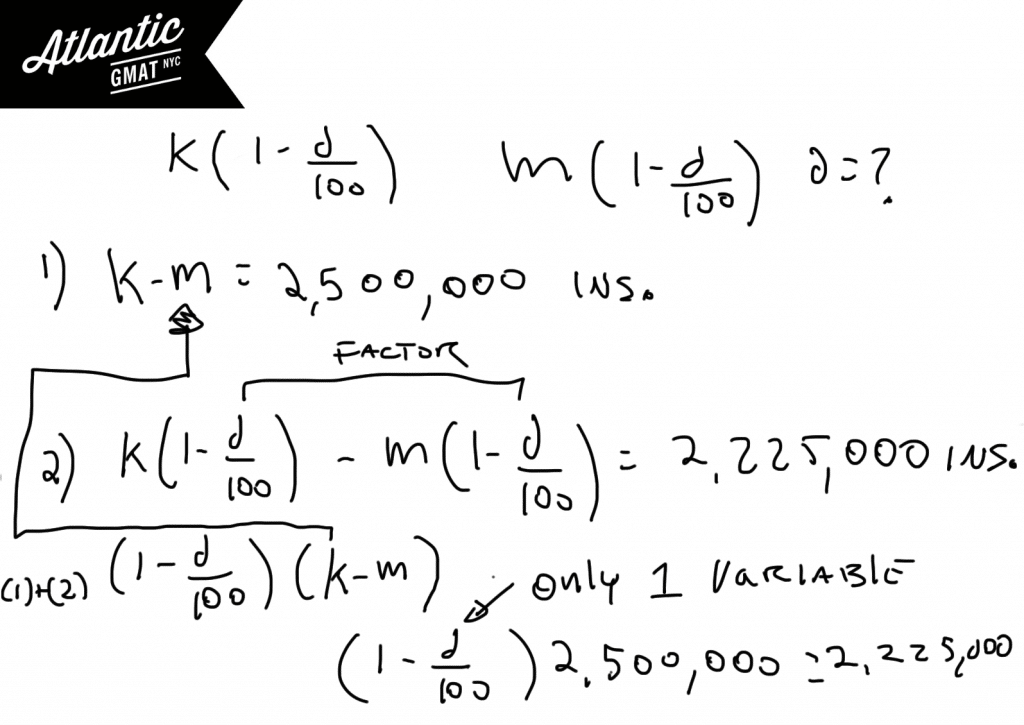# Data Sufficiency GMAT

The Data Sufficiency (DS) is unique to the GMAT Quant. It doesn't require solving for an answer but identifying whether there is enough information to solve for an answer hence the: Sufficiency. Many GMAT studiers start off in an adverse relation ship with the DS even though it actually requires a lot less work to solve Data Sufficiency questions than Problem Solving q's. Why? Well, you only have to identify whether you can get to an answer so often you don't have to crunch the numbers. The DS exemplifies what the GMAT is about: critical thinking.

## Data Sufficiency GMAT Example

If the total revenue at Company K and Company M decreased by d% from 2001 to 2003 what is the value of d?

(1) In 2001 Company K earned 2,500,000 more revenue than Company M earned.

(2) In 2003 Company K earned 2,225,000 more revenue than Company M earned.

The Data Sufficiency answer choices are always the same so: memorize them! No excuse for being foggy on DS answers. This is a "counting equations"/"system of equations" style DS question so:

1. Define the initial equation and identify how many variables you have.
2. Define each statement and see whether you end up with a 1:1 ratio of equations to variables. If that's the case, unless something odd is happening, you will be able to solve for the any of the variables in the system.

You will end up with two equations and three variables. Unless there is some special "perfect fit" happening you will be unable to solve for d. There happens to be a perfect fit if you substitute in for k-m. Once you do that you'll just have one variable and one equation and will be able to solve. So the answer is C, you can solve with both statements together. Here's the diagram:## How to study for GMAT Data Sufficiency?

-The DS is all about set-up. Most people go wrong by barreling into the details before having a handle on the big picture.

-Find the question behind the question. Often, making GMAT Data Sufficiency easy is about redefining the question. That then puts everything else into focus.

-Remember that DS is about testing sufficiency. Save time by analyzing sufficiency rather than solving for an answer that you don't need. That doesn't mean: be lazy. Be proactive setting things up, organizing information, defining the initial equation, and finding the question behind the question but of course save time and energy by only doing what is necessary in terms of crunching the numbers.

-Sometimes testing numbers is a fantastic strategy but sometimes it's awful and will leave you horribly distressed. Algebraic solutions can snap everything into place but they can also collapse around you like a prison. The point is: there's no one way of doing things. Be flexible. Sometimes smart numbers will uncover the answer while other times algebra will click.

# CONTACT

Atlantic GMAT

405 East 51st St.

NY, NY 10022

(347) 669-3545

info@AtlanticGMAT.com